GMAT Math : Arithmetic

Example Questions

Example Question #21 : Dsq: Understanding The Properties Of Integers

Does the integerhave at least 4 different positive prime factors?

(1)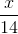is an integer.

(2)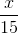is an integer.

BOTH statements TOGETHER are sufficient to answer the question, but NEITHER statement ALONE is sufficient.

EACH statement ALONE is sufficient to answer the question.

Statement 2 ALONE is sufficient to answer the question, but the other statement alone is not sufficient.

Statement 1 ALONE is sufficient to answer the question, but the other statement alone is not sufficient.

BOTH statements TOGETHER are not sufficient to answer the question.

BOTH statements TOGETHER are sufficient to answer the question, but NEITHER statement ALONE is sufficient.

Explanation:

(1) This statement tells us that 2 and 7 are prime factors of. This information is not sufficient.

(2) This statement tells us that 3 and 5 are prime factors of. this information is not sufficient.

Considering both (1) and (2), we have four positive prime factors ofbetween them.

Example Question #22 : Dsq: Understanding The Properties Of Integers

What is the remainder when the two digit, positive integeris divided by 3?

(1)  The sum of the digits ofis 3.

(2)  The difference of the digits is 3.

A. Statement (1) ALONE is sufficient, but statement (2) alone is not sufficient.

D. EACH statement ALONE is sufficient.

C. BOTH statements TOGETHER are sufficient, but NEITHER statement ALONE is sufficient.

E. Statements (1) and (2) TOGETHER are NOT sufficient.

B. Statement (2) ALONE is sufficient, but statement (1) alone is not sufficient.

A. Statement (1) ALONE is sufficient, but statement (2) alone is not sufficient.

Explanation:

For statement (1), there are 3 possible two digit positive integers: 30, 12, 21. The remainder when these numbers are divided by 3 is 0. Therefore, statement (1) is sufficient. For statement (2), there are lots of different combinations of integers that have different remainders when divided by 3. For example, the remainder of 30 is 0, but the remainder of 14 is 2. Therefore, statement (2) is insufficient.

Example Question #23 : Dsq: Understanding The Properties Of Integers,, andare integers. Is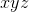odd?

(1)is a prime number and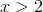(2)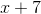is odd

Statement (1) ALONE is sufficient, but statement (2) alone is not sufficient.

Statements (1) and (2) TOGETHER are NOT sufficient.

BOTH statements TOGETHER are sufficient, but NEITHER statement ALONE is sufficient.

EACH statement ALONE is sufficient.

Statement (2) ALONE is sufficient, but statement (1) alone is not sufficient.

Statement (2) ALONE is sufficient, but statement (1) alone is not sufficient.

Explanation:

Statement (1) tells us thatis an odd number. Since we don’t know ifandare odd numbers, we cannot decide the sign of.

Statement (2) tells us thatis an even number, since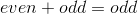. When we do the multiplication, the product will be even if one or more of the integers are even. So with statement (2), we know for sure thatis even.

Example Question #24 : Dsq: Understanding The Properties Of Integers

Is a whole number a perfect square integer?

Statement 1: It falls between 110 and 120 inclusive.

Statement 2: Its last digit is a 7.

Statement 2 ALONE is sufficient to answer the question, but Statement 1 ALONE is NOT sufficient to answer the question.

BOTH statements TOGETHER are insufficient to answer the question.

BOTH statements TOGETHER are sufficient to answer the question, but NEITHER statement ALONE is sufficient to answer the question.

EITHER statement ALONE is sufficient to answer the question.

Statement 1 ALONE is sufficient to answer the question, but Statement 2 ALONE is NOT sufficient to answer the question.

EITHER statement ALONE is sufficient to answer the question.

Explanation:

There are no perfect square integers between 110 and 120 inclusive: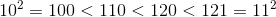Also, all perfect squares end in 0, 1, 4, 5, 6, or 9, depending on the last digit of the number being squared.

From ether statement alone, it therefore follows that the number is not a perfect square integer.

Example Question #25 : Dsq: Understanding The Properties Of Integers

Is a whole number a perfect square?

Statement 1: Its last digit is 9.

Statement 2: It falls between 160 and 170 inclusive.

EITHER statement ALONE is sufficient to answer the question.

BOTH statements TOGETHER are sufficient to answer the question, but NEITHER statement ALONE is sufficient to answer the question.

BOTH statements TOGETHER are insufficient to answer the question.

Statement 1 ALONE is sufficient to answer the question, but Statement 2 ALONE is NOT sufficient to answer the question.

Statement 2 ALONE is sufficient to answer the question, but Statement 1 ALONE is NOT sufficient to answer the question.

BOTH statements TOGETHER are sufficient to answer the question, but NEITHER statement ALONE is sufficient to answer the question.

Explanation:

Statement 1 alone does not answer the question, since some integers ending in 9 are perfect squares (9, 49) and some are not (29, 39).

Statement 2 alone does not answer the question, since of the numbers in that range, only 169 is a perfect square (of 13).

From both statements together, however, it follows that the number in question is 169, which, as just stated, is the square of 13.

Example Question #21 : Properties Of Integers

I am thinking of two distinct prime numbers. What are they?

Statement 1: Both are between 50 and 60.

Statement 2: Their sum is 112.

Statement 1 ALONE is sufficient to answer the question, but Statement 2 ALONE is NOT sufficient to answer the question.

BOTH statements TOGETHER are sufficient to answer the question, but NEITHER statement ALONE is sufficient to answer the question.

EITHER statement ALONE is sufficient to answer the question.

BOTH statements TOGETHER are insufficient to answer the question.

Statement 2 ALONE is sufficient to answer the question, but Statement 1 ALONE is NOT sufficient to answer the question.

Statement 1 ALONE is sufficient to answer the question, but Statement 2 ALONE is NOT sufficient to answer the question.

Explanation:

Statement 1 is a giveaway; the only prime numbers between 50 and 60 are 53 and 59.

Statement 2 is not a giveaway, though, since there are at least two sums of primes equal to 112: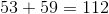and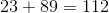Example Question #27 : Dsq: Understanding The Properties Of Integers

Is a positive integera prime number or a composite number?

Statement 1: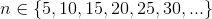Statement 2: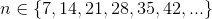BOTH statements TOGETHER are insufficient to answer the question.

EITHER statement ALONE is sufficient to answer the question.

BOTH statements TOGETHER are sufficient to answer the question, but NEITHER statement ALONE is sufficient to answer the question.

Statement 2 ALONE is sufficient to answer the question, but Statement 1 ALONE is NOT sufficient to answer the question.

Statement 1 ALONE is sufficient to answer the question, but Statement 2 ALONE is NOT sufficient to answer the question.

BOTH statements TOGETHER are sufficient to answer the question, but NEITHER statement ALONE is sufficient to answer the question.

Explanation:

From Statement 1 it follows thatis a multiple of 5, but it does not answer the question by itself; 5 is prime, but the other multiples of 5 are composite. Similarly, from Statement 2 it follows thatis a multiple of 7, but, 7 is prime and the other multiples of 7 are composite.

If we can assume both statements, then, since 5 and 7 are relatively prime, it follows thatis a multiple of both 5 and 7; sincenow has at least four factors (1,5, 7, and 35),is composite.

Example Question #28 : Dsq: Understanding The Properties Of Integers

Is the product of three positive integers divisible by 4?

Statement 1: The three integers are consecutive.

Statement 2: Two of the integers are even.

BOTH statements TOGETHER are insufficient to answer the question.

Statement 2 ALONE is sufficient to answer the question, but Statement 1 ALONE is NOT sufficient to answer the question.

BOTH statements TOGETHER are sufficient to answer the question, but NEITHER statement ALONE is sufficient to answer the question.

Statement 1 ALONE is sufficient to answer the question, but Statement 2 ALONE is NOT sufficient to answer the question.

EITHER statement ALONE is sufficient to answer the question.

Statement 2 ALONE is sufficient to answer the question, but Statement 1 ALONE is NOT sufficient to answer the question.

Explanation:

Statement 1 is not enough to answer the question. For example, the integers can be 3, 4, and 5, the product of which is 60, which is divisible by 4; or, the integers can be 5, 6, and 7, the product of which is 210, which is not divisible by 4.

From Statement 2, however, it can be inferred that the product is divisible by 4. If two of the integers are even, the two can be written as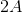and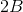for some integers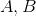. If we call the third integer, the product is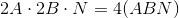, and the product must be divisible by 4.

Example Question #1011 : Data Sufficiency Questions

Is the product of three integers divisible by 6?

Statement 1: The three integers are consecutive.

Statement 2: One integer is even and two are odd.

EITHER statement ALONE is sufficient to answer the question.

BOTH statements TOGETHER are insufficient to answer the question.

Statement 1 ALONE is sufficient to answer the question, but Statement 2 ALONE is NOT sufficient to answer the question.

Statement 2 ALONE is sufficient to answer the question, but Statement 1 ALONE is NOT sufficient to answer the question.

BOTH statements TOGETHER are sufficient to answer the question, but NEITHER statement ALONE is sufficient to answer the question.

Statement 1 ALONE is sufficient to answer the question, but Statement 2 ALONE is NOT sufficient to answer the question.

Explanation:

Any group of three consecutive integers must include at least one multiple of 3 and at least one even number (multiple of 2). Therefore, if Statement 1 is assumed, 2 and 3 both divide the product of the integers and, subsequently, so does 6.

Statement 2 is not enough, however - for example, the product of 3, 4, and 5 is 60, a multiple of 3, but the product of 4, 5, and 7 is 140, not a multiple of 3.

Example Question #30 : Dsq: Understanding The Properties Of Integers

The triangle, the square, and the circle are replaced with three (not necessarily different) digits to form a six-digit integer.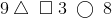Is 4 a factor of this integer?

Statement 1: The square and the triangle are replaced by the same digit.

Statement 2: The circle is replaced by a 5.

Statement 1 ALONE is sufficient to answer the question, but Statement 2 ALONE is NOT sufficient to answer the question.

Statement 2 ALONE is sufficient to answer the question, but Statement 1 ALONE is NOT sufficient to answer the question.

BOTH statements TOGETHER are insufficient to answer the question.

EITHER statement ALONE is sufficient to answer the question.

BOTH statements TOGETHER are sufficient to answer the question, but NEITHER statement ALONE is sufficient to answer the question.